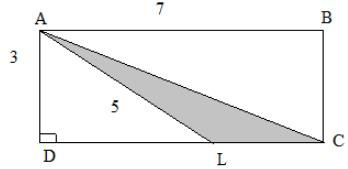Courses

# What is the area of triangle ALC in the figure given below?a)2.5b)3c)3.5d)4e)4.5Correct answer is option 'E'. Can you explain this answer? Related Test: Test: Problem Solving- 1

## GMAT Question

By SAVI MISHRA · Sep 26, 2020 ·GMAT
Venky Mouni answered Jul 25, 2019
In the figure height isAL
then y we take 3 as height

Indu Bahl answered Jul 21, 2019
First of all, we need to find LC, but for that, we need to find DL. We know that AB= DC = 7.
So, LC = DC- DL
Now, DL = √(5^2- 3^2 )= 4 (using Pythagoras Theorem)
So, LC = 7-4 = 3
Therefore, Area = (1/2)(Base)(Altitude) = (0.5)(3)(3) = 4.5